# Year 6 Printable primary worksheets

All of our Year 6 resources come complete with answer sheets to save time for busy teachers and parents. Input your child’s scores and keep track of your child's progress.

Filters

### 658 results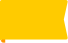New

### Two-step function machines

Students are asked to follow a rule and calculate the output for each input.

Age: 10 - 11
1
2
3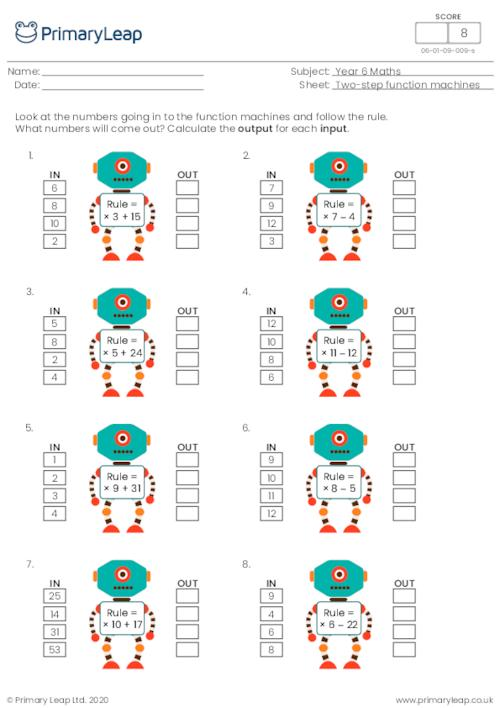New

### Write the rule (2 operations)

Students are asked to look at the numbers going in and the numbers coming out of the function machines, then write the rule in each function machine. Each rule is made up of 2 operations e.g. × then +.

Age: 10 - 11
1
2
3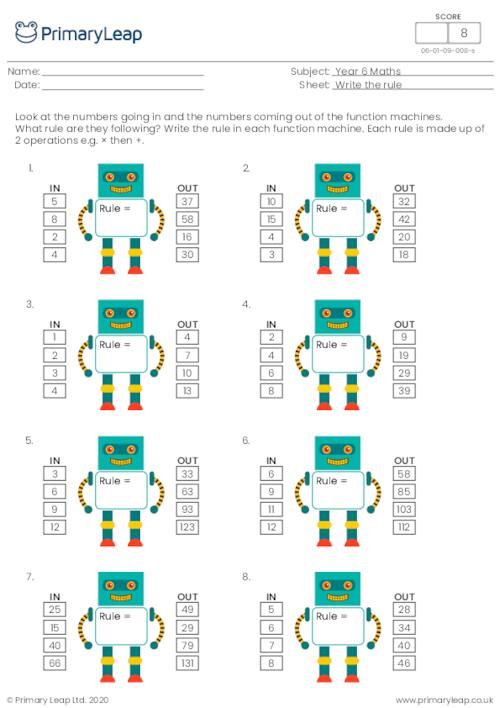New

### Balancing equations - Multiplication and division

Students are asked to work out the sums by finding the value of each letter.

Age: 10 - 11
1
2
3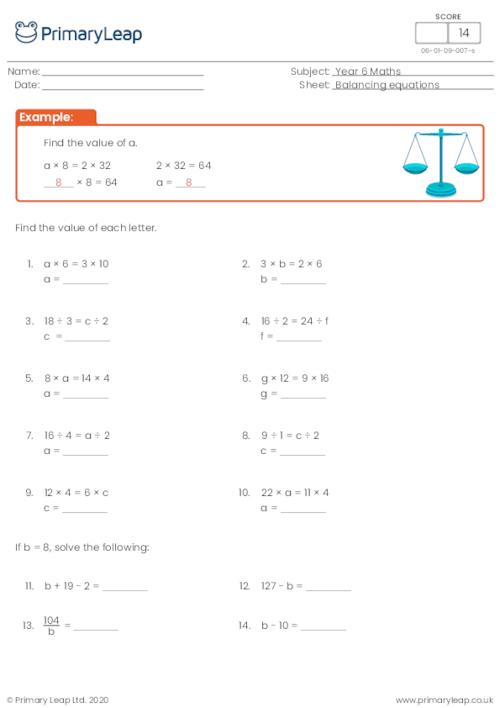New

### Finding a rule

Students are asked to complete the number sequences and find a rule for each sequence.

Age: 10 - 11
1
2
3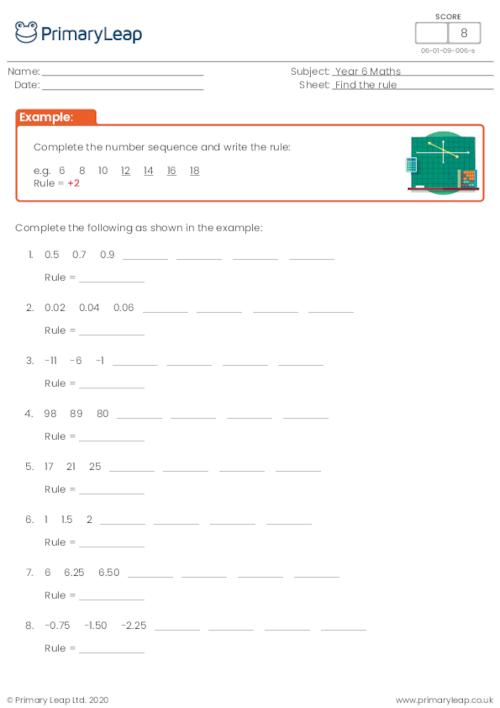New

### Number sequences

Students complete the number sequences and then follow the rule to give the first 5 numbers of each sequence.

Age: 10 - 11
1
2
3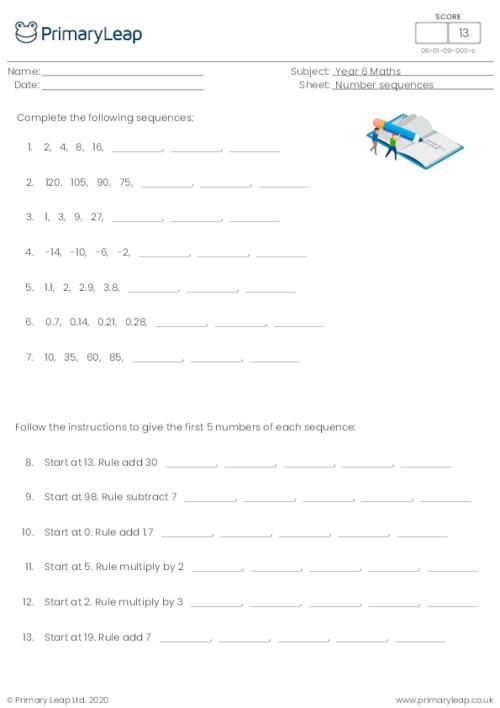New

### Balancing equations - Addition and subtraction

Students are asked to work out the sums by finding the value of each letter.

Age: 10 - 11
1
2
3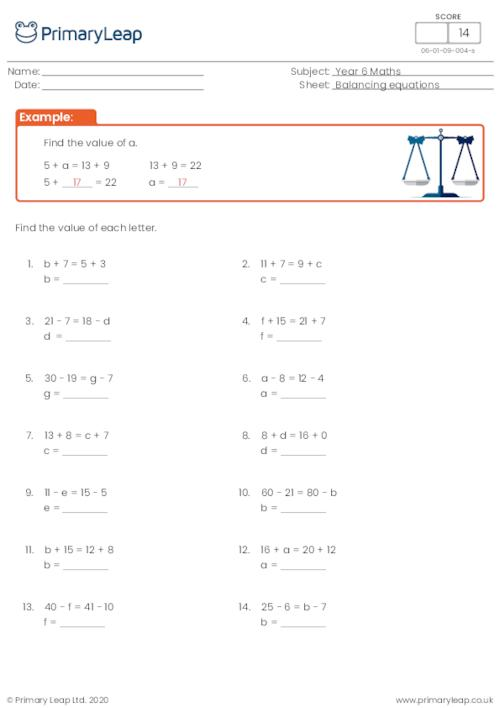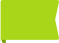Free

### Write the equation

Students are asked to write the equation relating the input (x) to the output (y), then fill in the missing boxes to complete each table.

Age: 10 - 11
1
2
3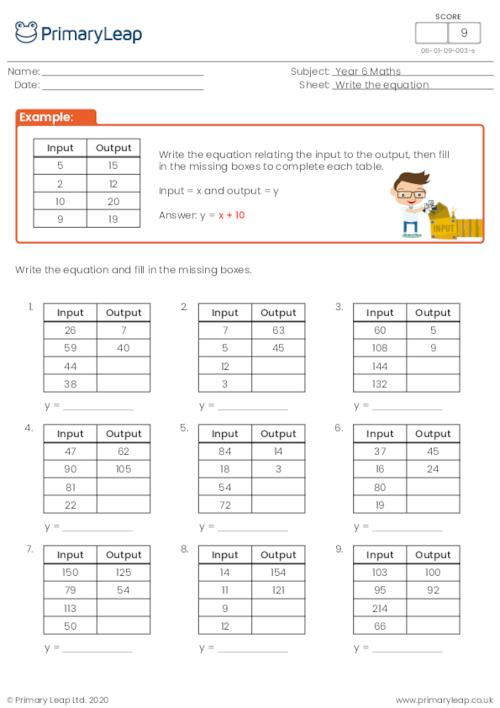New

### Calculate the expression

Students are asked to complete the sums when the value of a letter is given e.g. a = 9, b = 6 and c = 12.

Age: 10 - 11
1
2
3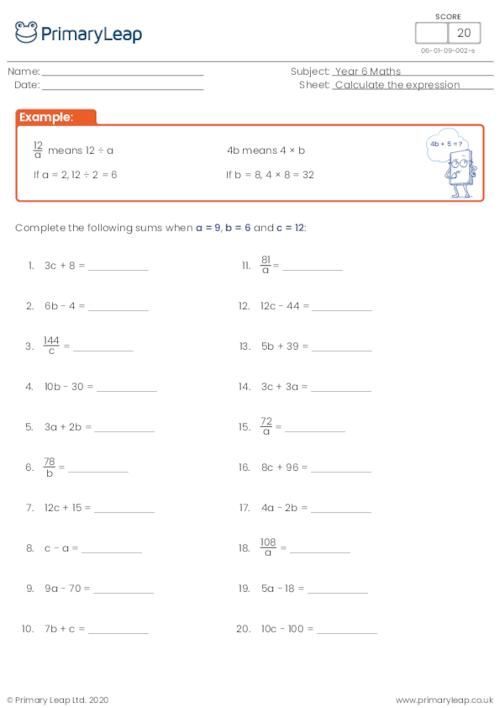New

### Introducing algebra

Students are asked to read through the sums and find the value of 'x'.

Age: 10 - 11
1
2
3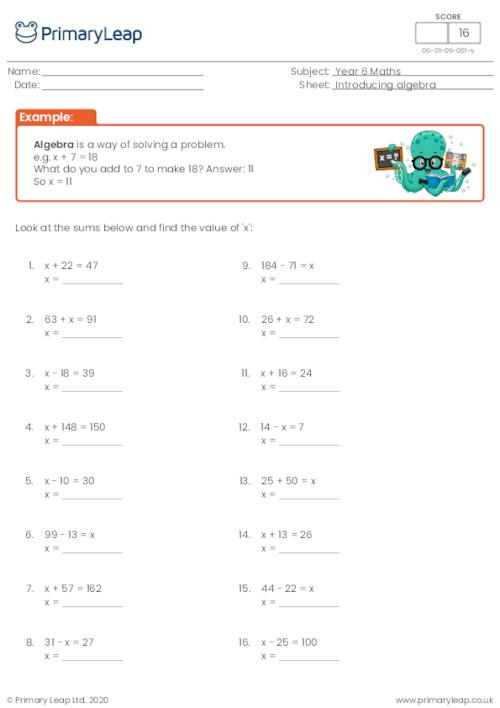New

### Algebraic expressions

Students are asked to write expressions using algebra.

Age: 10 - 11
1
2
3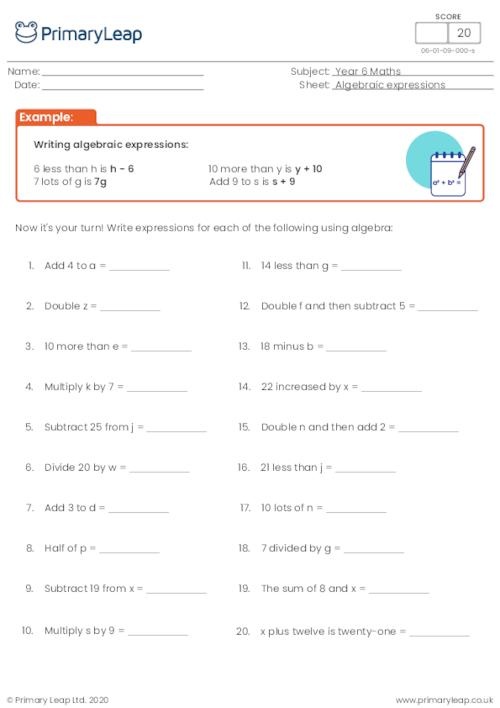New

### Parts of a circle

This maths resource asks students to identify the different parts of a circle: circle, radius and diameter.

Age: 10 - 11
1
2
3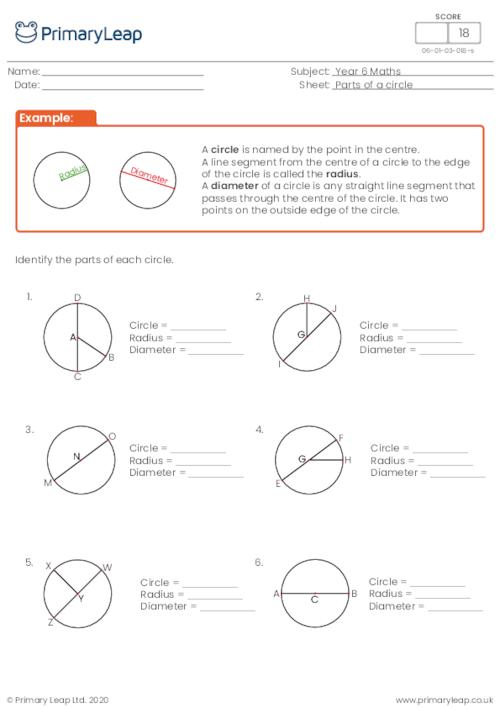New

### Classifying angles

Students label each angle with 'acute', 'obtuse' or 'reflex'.

Age: 10 - 11
1
2
3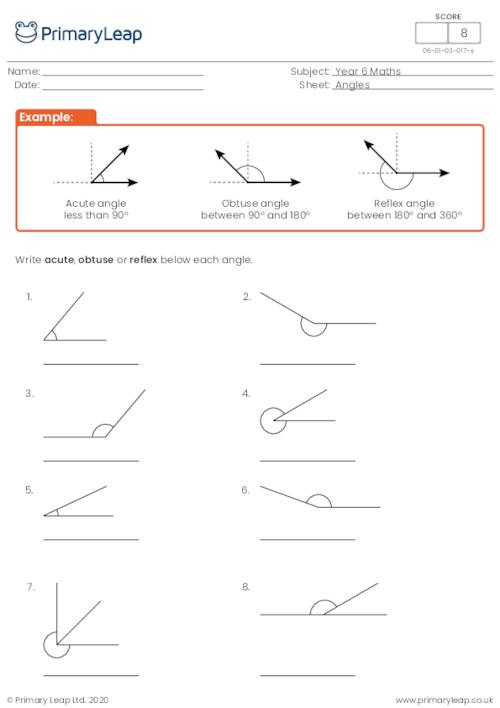New

### Net of a tetrahedron

Students make a tetrahedron by carefully following the instructions.

Age: 10 - 11
1
2
3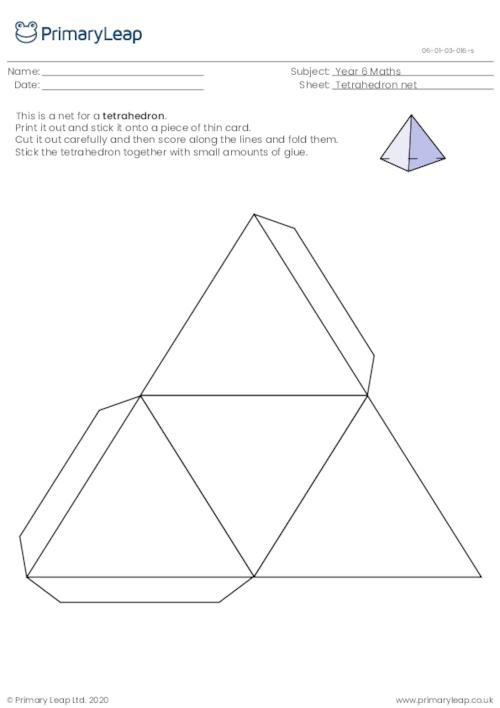New

### Converting units of weight

Students are asked to convert between tons, ounces and pounds.

Age: 10 - 11
1
2
3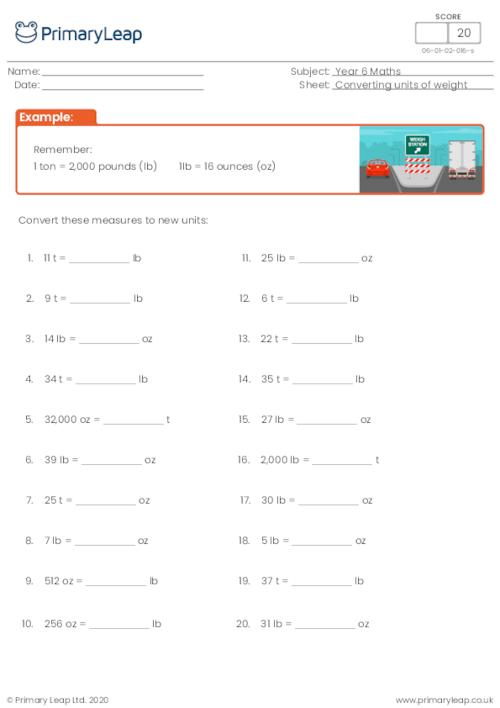New

### Converting between liquid volume units

Students practise converting liquid volume units between ounces, cups, pints, quarts and gallons.

Age: 10 - 11
1
2
3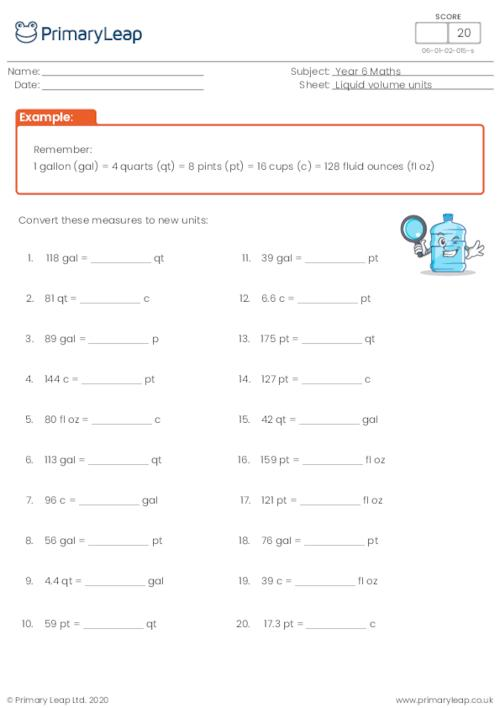New

### Converting metric units of weight

Students are asked to convert the measurements from kg to g and vice versa.

Age: 10 - 11
1
2
3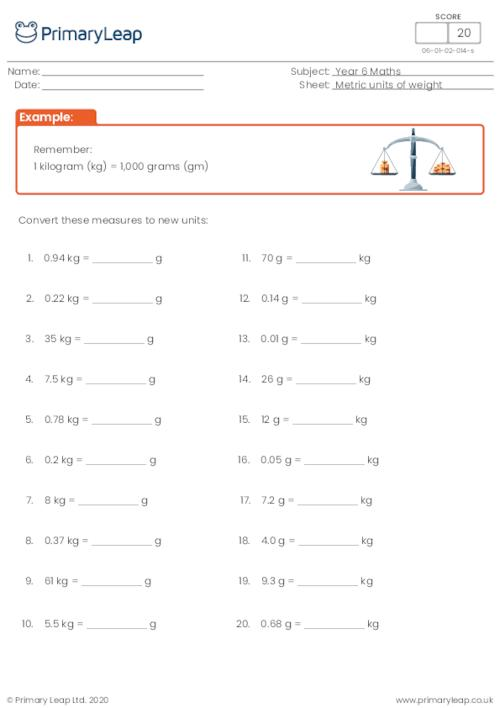New

### Area of a parallelogram

Students are asked to find the area of the parallelogram.

Age: 10 - 11
1
2
3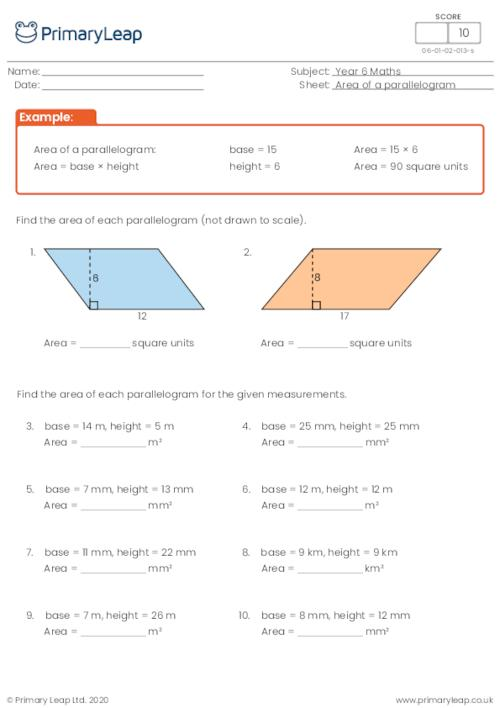New

### Area of a triangle

Students are asked to find the area of each triangle.

Age: 10 - 11
1
2
3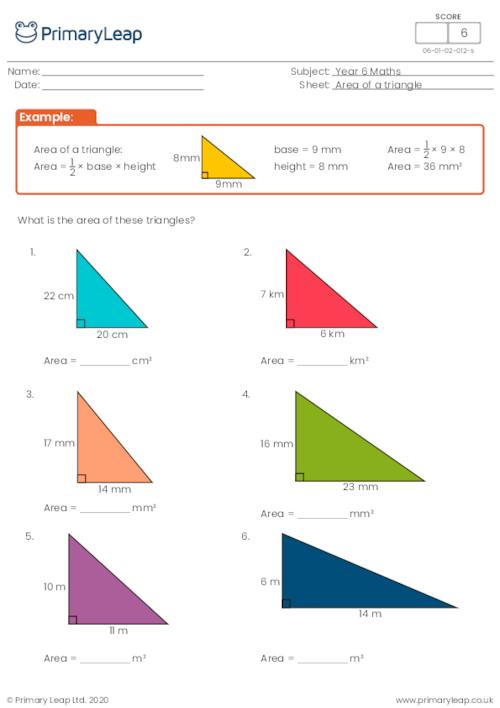New

### Converting between miles, yards and feet

Students practise converting between miles, yards and feet.

Age: 10 - 11
1
2
3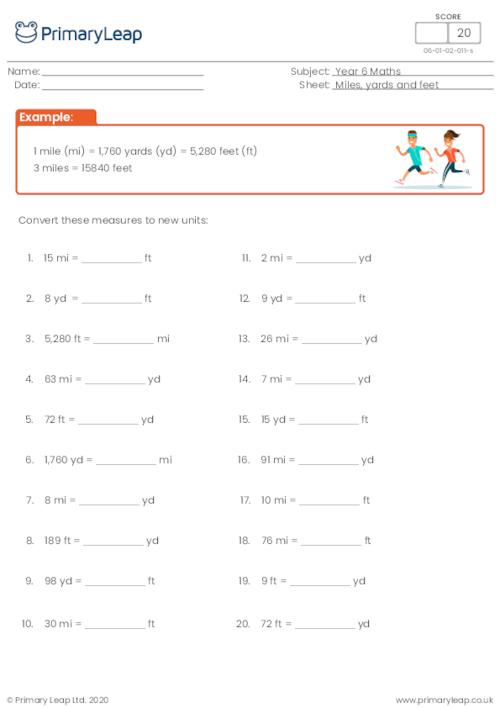New

### Estimating and converting metric units

Students estimate metric measures and convert smaller units of measure to larger units and vice versa.

Age: 10 - 11
1
2
3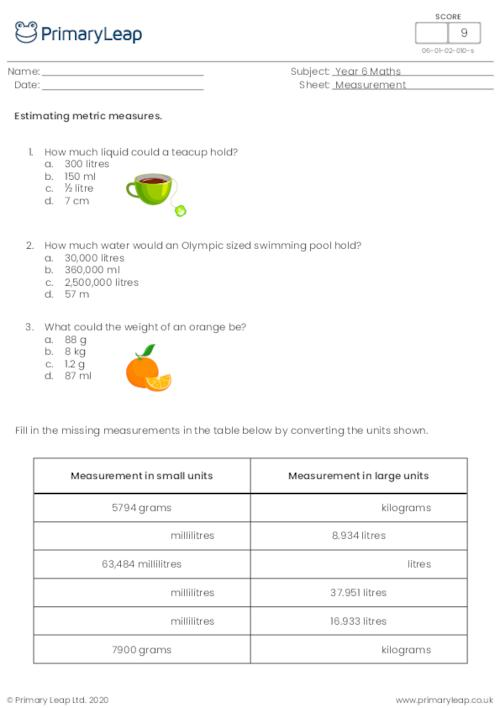New

### Converting metric units of length

Students fill in the table by converting to the units shown.

Age: 10 - 11
1
2
3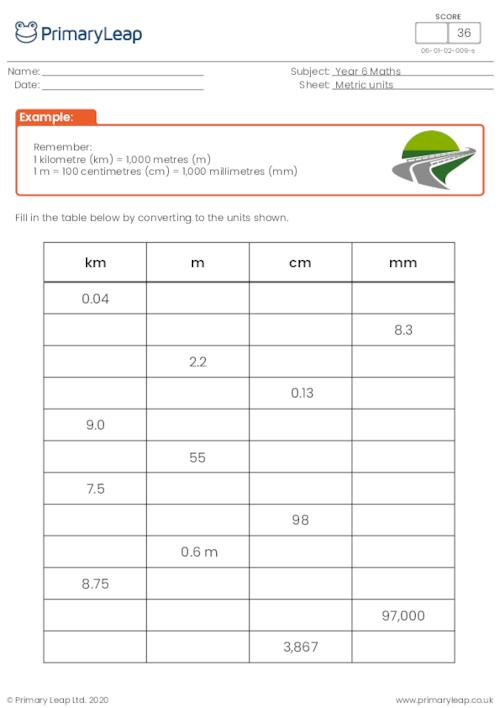New

### Metric units

Students choose the unit of measure that would be the most appropriate to measure the items

Age: 10 - 11
1
2
3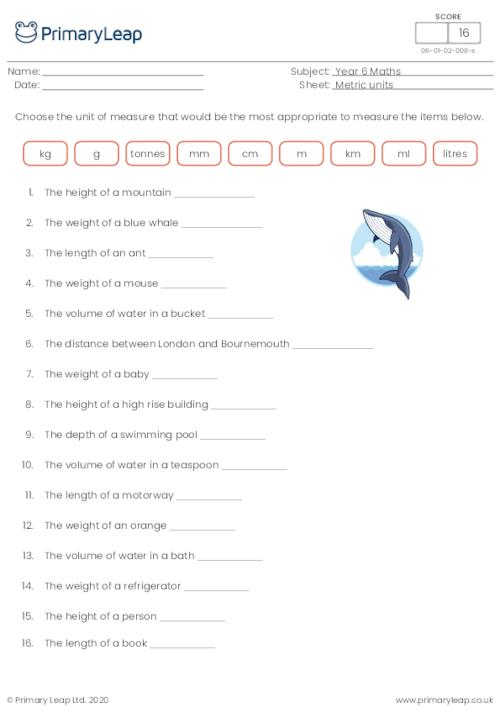New

### Multiplying fractions

Level 3 worksheet: Multiplying fractions with denominators between 2 and 20. Students multiply the fractions and give the answer in its simplest form.

Age: 10 - 11
1
2
3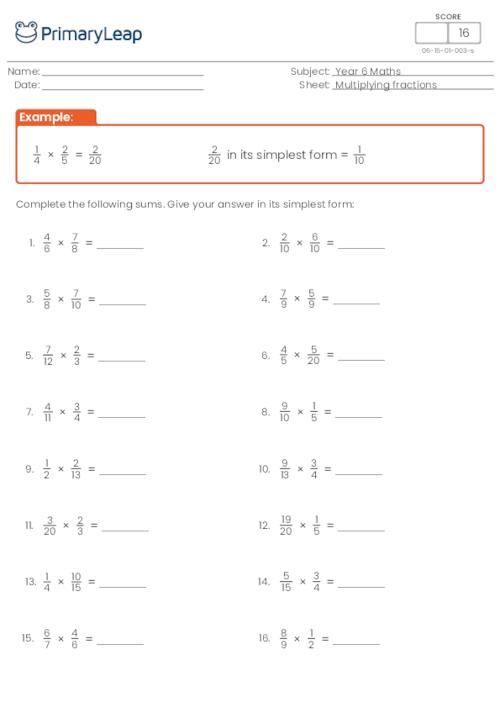New

### Multiplying fractions

Level 2 worksheet: Multiplying fractions with denominators between 2 and 12. Students multiply the fractions and give the answer in its simplest form.

Age: 10 - 11
1
2
3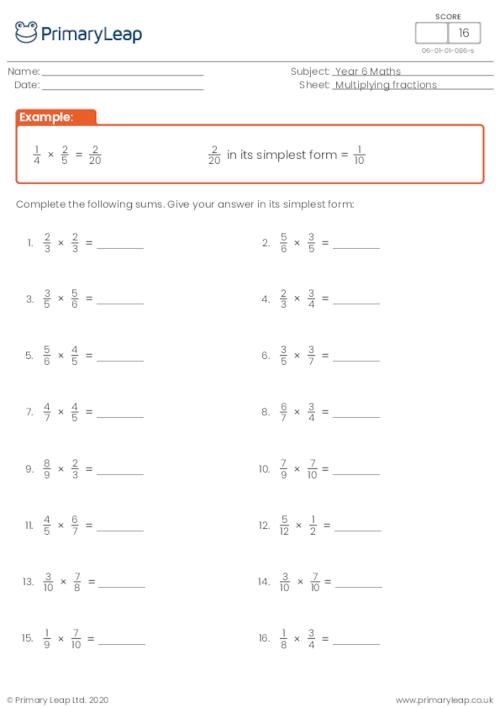New

### Multiplying fractions

Level 1 worksheet: Multiplying fractions with denominators between 2 and 8. Students multiply the fractions and give the answer in its simplest form.

Age: 10 - 11
1
2
3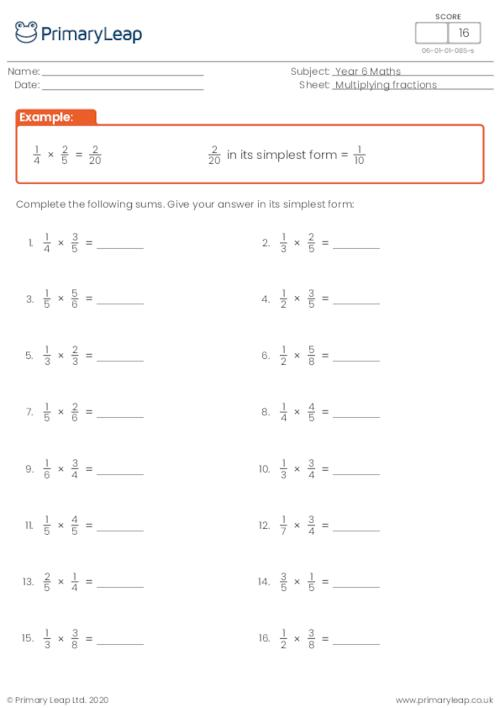New

### Subtracting fractions

This level 3 worksheet asks students to find the sum and write their answer in its simplest form.

Age: 10 - 11
1
2
3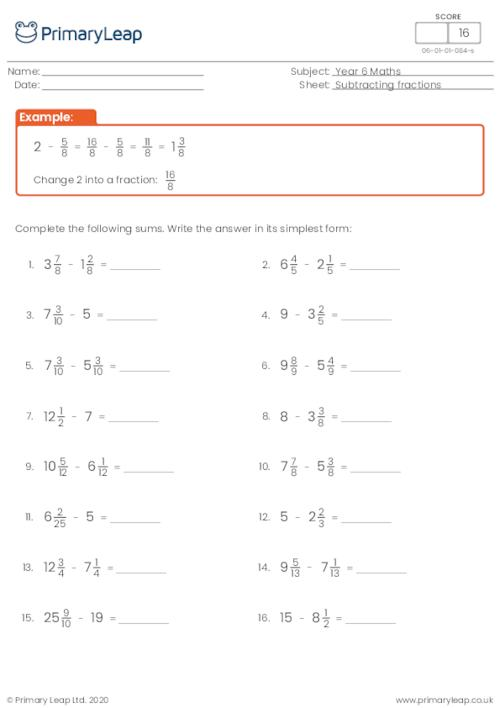New

### Subtracting fractions

This level 2 worksheet asks students to find the sum and write their answer in its simplest form.

Age: 10 - 11
1
2
3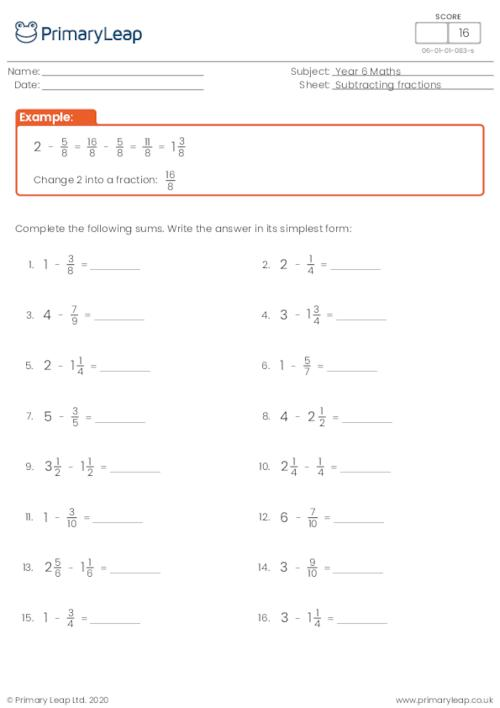New

### Subtracting fractions

This level 1 worksheet asks students to find the sum and write their answer in its simplest form.

Age: 10 - 11
1
2
3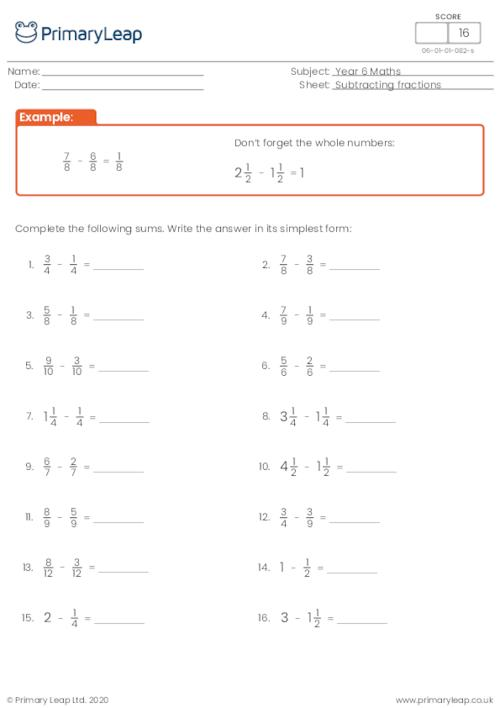New

This level 3 worksheet asks students to add the fractions and write their answer as a mixed fraction where necessary.

Age: 10 - 11
1
2
3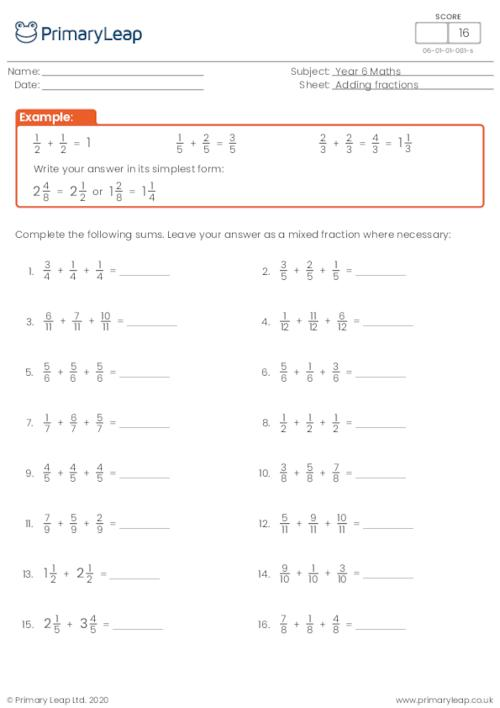New

This level 2 worksheet asks students to add the fractions and write their answer as a mixed fraction where necessary.

Age: 10 - 11
1
2
3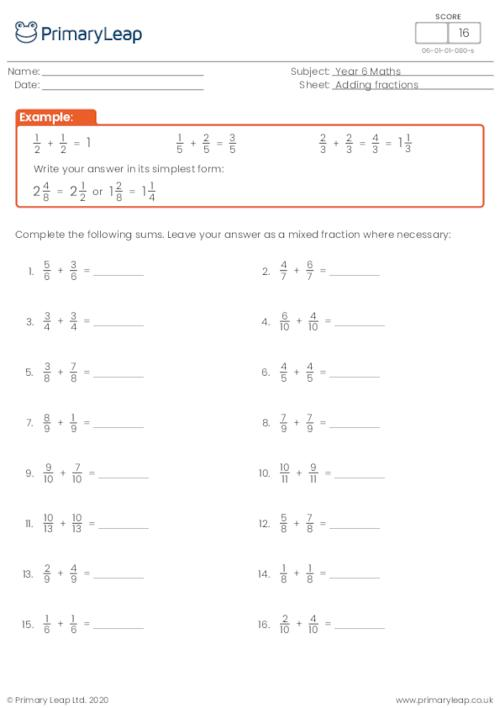New

This level 1 worksheet asks students to add the fractions and write their answer as a mixed fraction where necessary.

Age: 10 - 11
1
2
3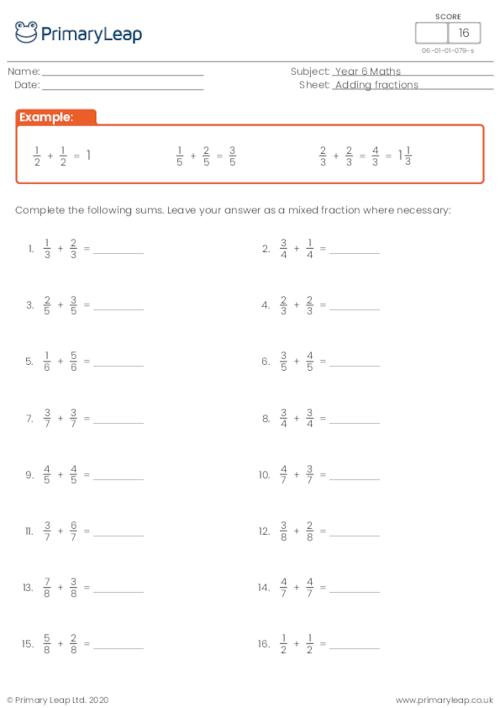New

### Converting improper fractions to mixed numbers

This level 3 (harder) worksheet asks students to change the improper fractions to mixed fractions and then from mixed fractions to improper fractions.

Age: 10 - 11
1
2
3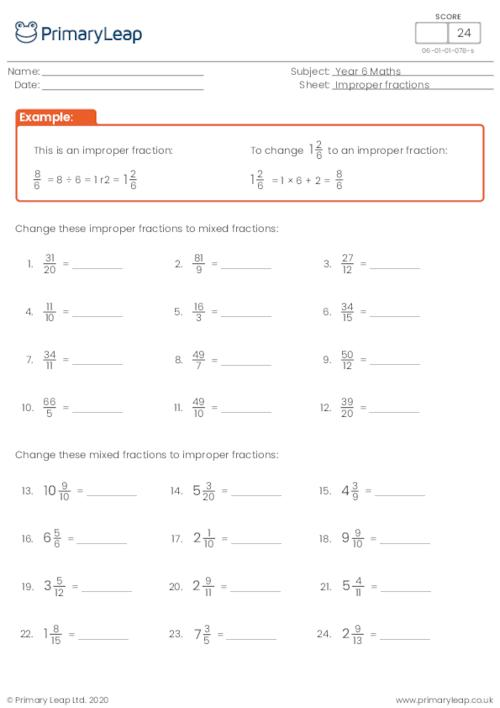New

### Converting improper fractions to mixed numbers

This level 2 worksheet asks students to change the improper fractions to mixed fractions and then from mixed fractions to improper fractions.

Age: 10 - 11
1
2
3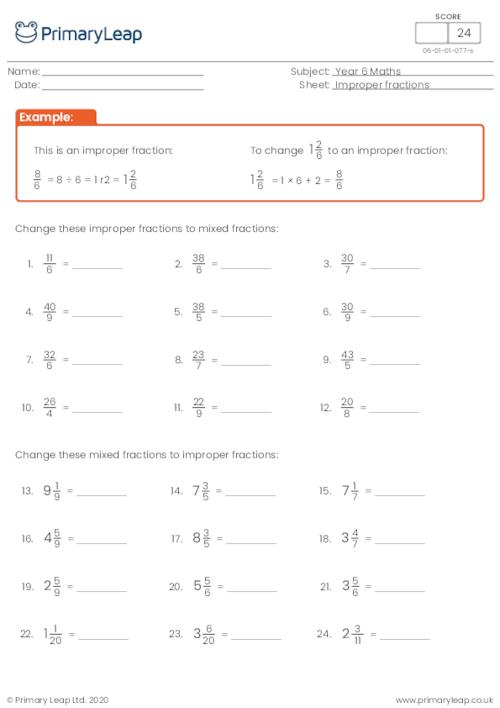New

### Converting improper fractions to mixed numbers

This worksheet asks students to change the improper fractions to mixed fractions and then from mixed fractions to improper fractions.

Age: 10 - 11
1
2
3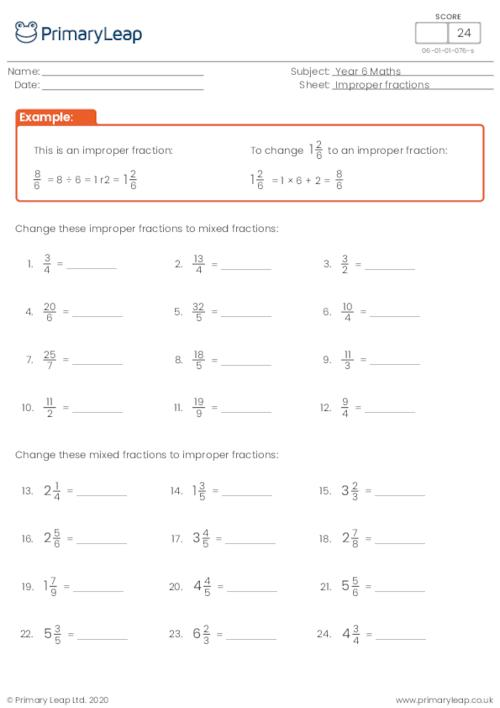### Word problems with multiplying and dividing

Students solve the word problems involving multiplying and dividing.

Age: 10 - 11
1
2
3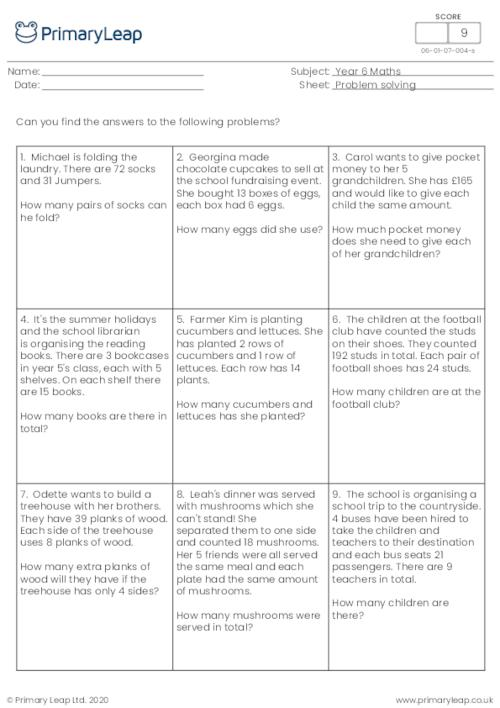### Word problems with 4 operations

Students solve the word problems involving the 4 basic operations: Adding, subtracting, multiplying and dividing.

Age: 10 - 11
1
2
3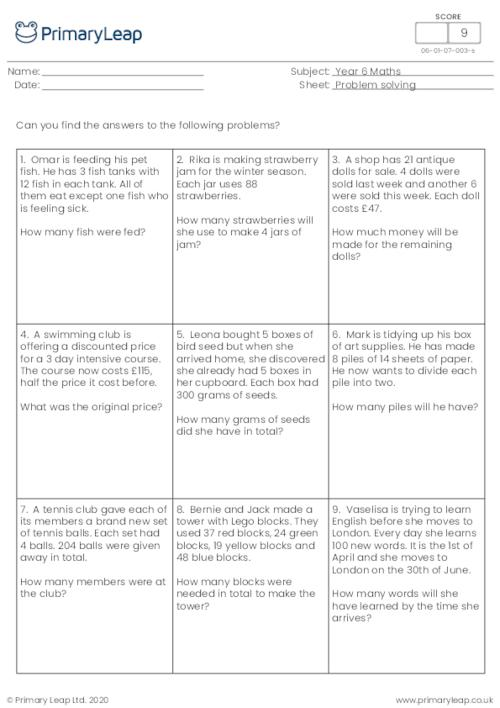### Identify prime numbers

Students are asked to circle the prime numbers out of the numbers given.

Age: 10 - 11
1
2
3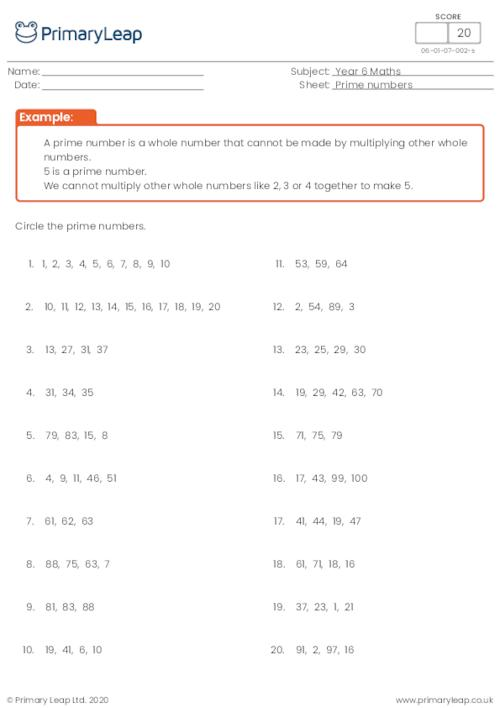### Find the factor pairs of a number

Students are asked to find the factor pairs of each given number.

Age: 10 - 11
1
2
3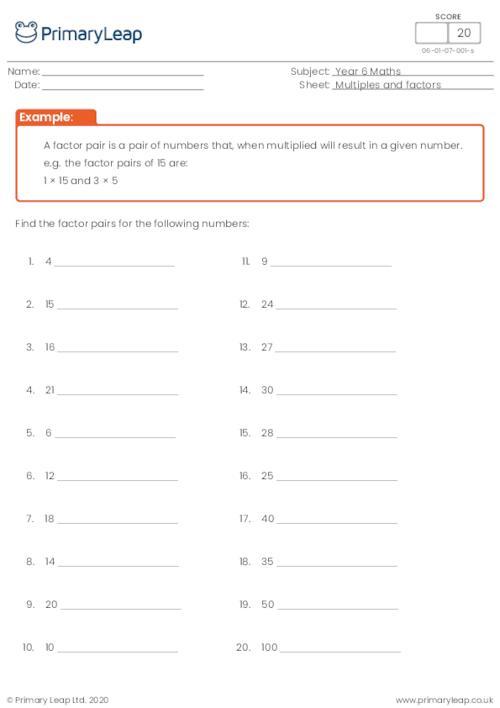### Identify multiples and factors

Students are asked to find the common factors between the multiples given.

Age: 10 - 11
1
2
3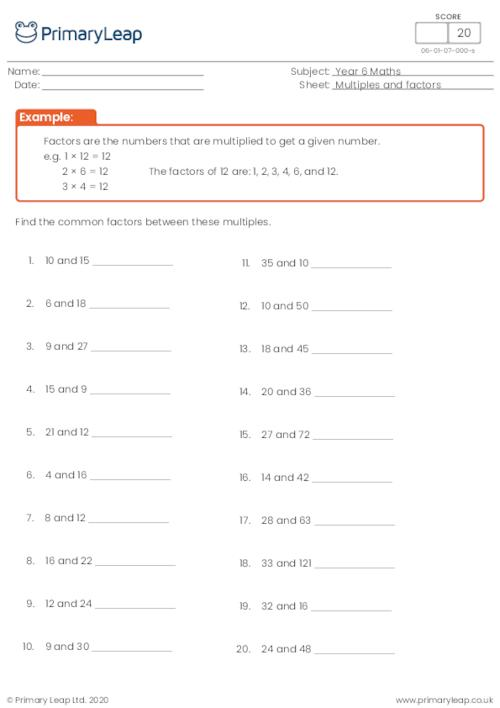### Rounding to the nearest 1000

Students round the numbers to the nearest 1,000.

Age: 10 - 11
1
2
3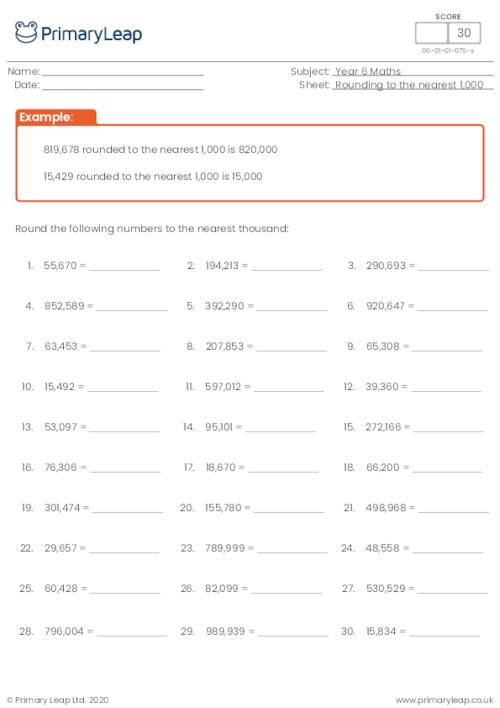### Rounding to the nearest 100

Students round the numbers to the nearest 100.

Age: 10 - 11
1
2
3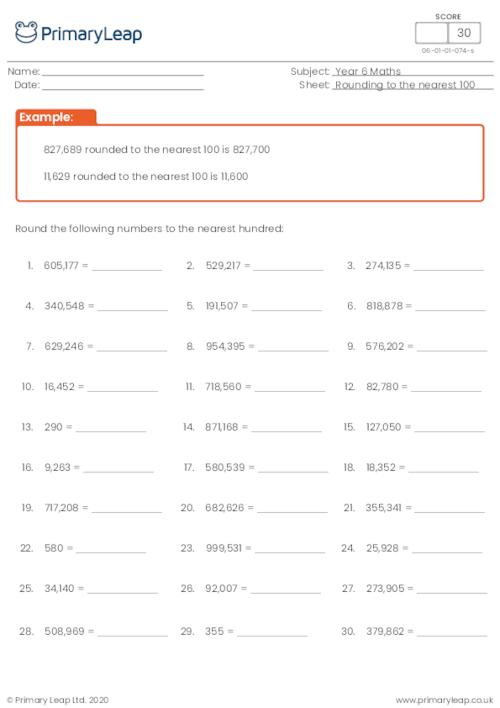### Rounding to the nearest 10

Students round the numbers to the nearest 10.

Age: 10 - 11
1
2
3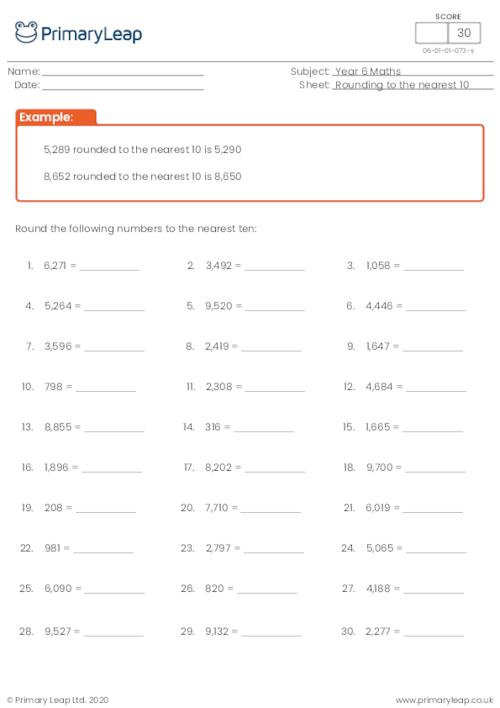### Missing value - 6-digit numbers (2)

Students fill in the missing value to complete the 6-digit number.

Age: 10 - 11
1
2
3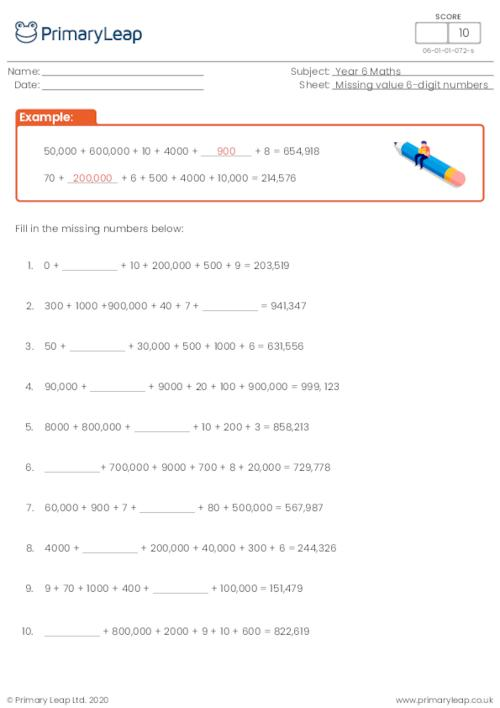### Missing value - 6-digit numbers (1)

Students fill in the missing value to complete the 6-digit number.

Age: 10 - 11
1
2
3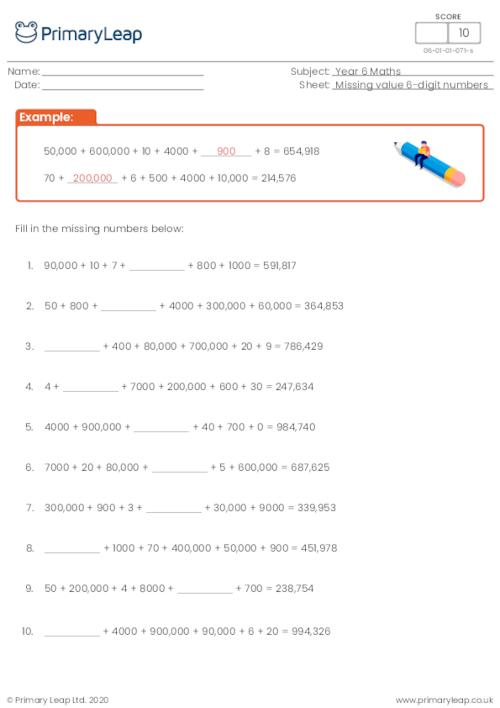### Missing value - 5-digit numbers (2)

Students fill in the missing value to complete the 5-digit number.

Age: 10 - 11
1
2
3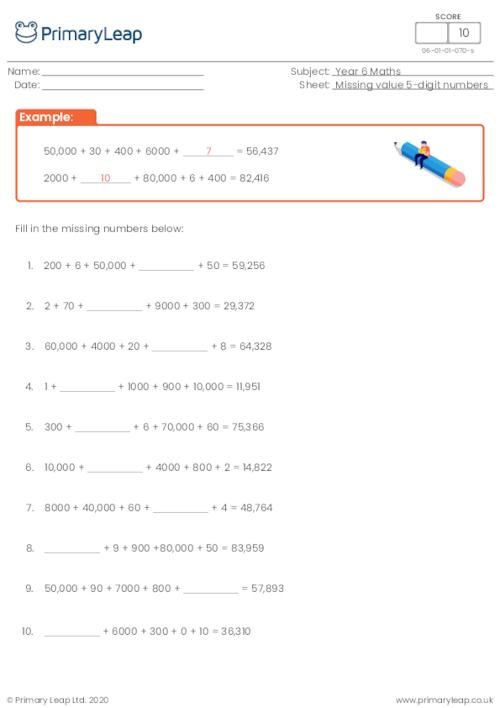### Missing value - 5-digit numbers (1)

Students fill in the missing value to complete the 5-digit number.

Age: 10 - 11
1
2
3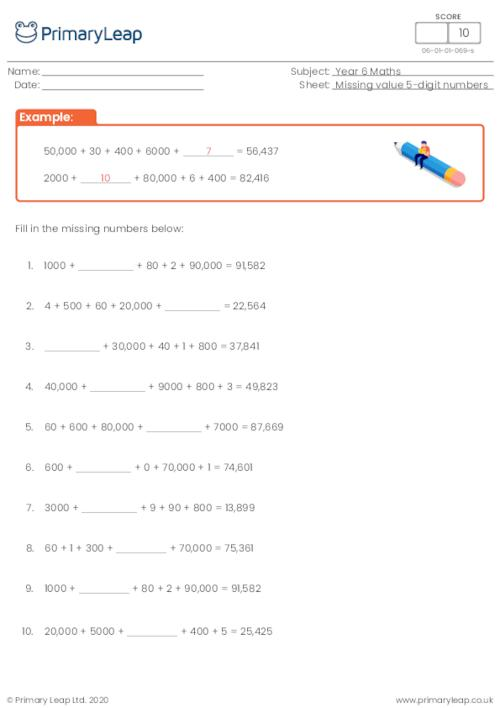### Place value - 6-digit numbers (2)

Students practise building a 6-digit number from the numbers given e.g. 749,175 = 700,000 + 40,000 + 9000 + 100 + 70 + 5

Age: 10 - 11
1
2
3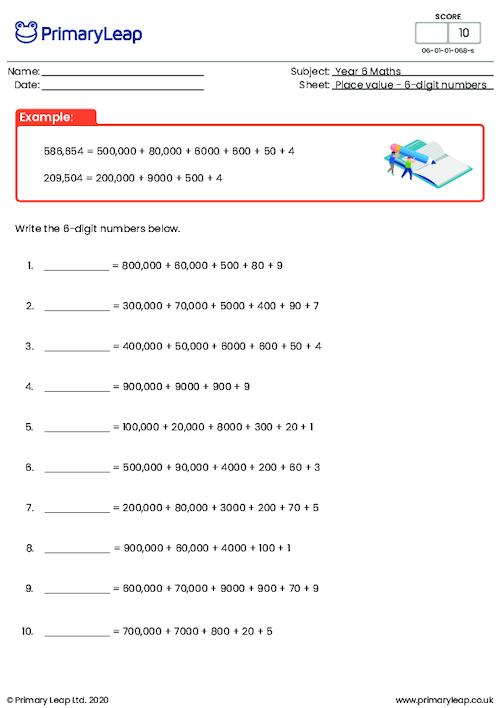### Place value - 6-digit numbers (1)

Students practise building a 6-digit number from the numbers given e.g. 749,175 = 700,000 + 40,000 + 9000 + 100 + 70 + 5

Age: 10 - 11
1
2
3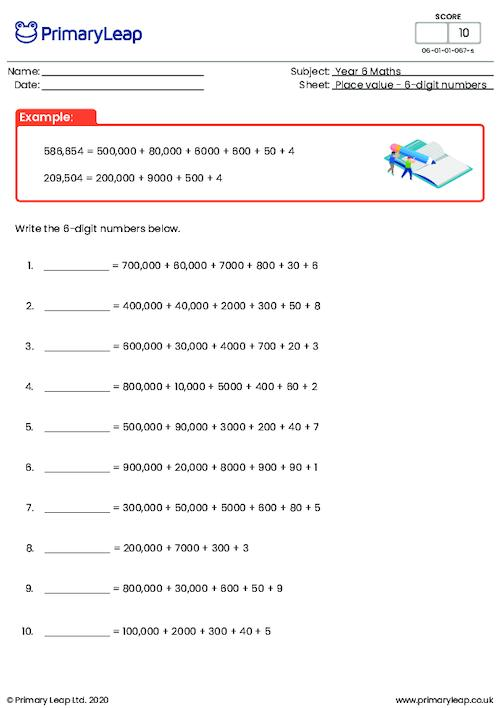of 14## Year 6 Worksheets - Created by teachers and loved by kids

Check out our ready-to-use Year 6 worksheets that can be used in the classroom or at home. Simply download or print and watch your child’s confidence grow with our easy to follow Year 6 printables.Learn

One of the best things about printable worksheets is that they make learning fun and easy. Explore our educational resources based on Year 6 and keep your children learning through these fun and engaging exercises.Progress

Our Year 6 printable worksheets are designed for progressive learning. Our engaging content enables a child to learn at their own speed and gain confidence quickly. When we help a child believe in themselves, we empower them for life.Improve